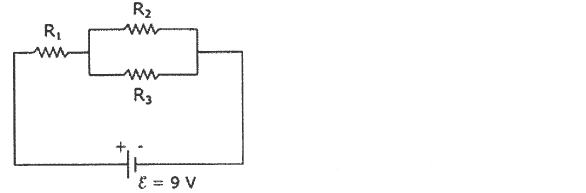Problem: The circuit in the sketch consists of three resistors and a battery with emf ε = 9.0 V and negligible internal resistance. R1 = 2.0 Ω, R2 = 12.0 Ω, and R3 = 4.0 Ω.What is the current flowing through each resistor? I1 = _______________I2 = _______________I3 = _______________

FREE Expert Solution
86% (154 ratings)
Problem Details

The circuit in the sketch consists of three resistors and a battery with emf ε = 9.0 V and negligible internal resistance. R1 = 2.0 Ω, R2 = 12.0 Ω, and R3 = 4.0 Ω.

What is the current flowing through each resistor?

I1 = _______________

I2 = _______________

I3 = _______________